# （三）提升树模型：Lightgbm原理深入探究

LightGBM的资料网上很多，但是讲解得都很浅，大部分都是从官方文档摘抄过来的，没有深究。

2.1 速度和内存的优化

2.2 准确率的优化

2.2.1 Leaf-wise (Best-first) 的决策树生长策略

2.2.2 类别特征值的最优分割

2.3 并行学习的优化

2.3.1 特征并行

2.3.2 数据并行

2.3.3 投票并行

3.1 如果改变数据集的特征的顺序，为什么训练的结果却不一样？

3.2 在custom objective中Hessian的作用是什么呢？

3.3 scale_pos_weight参数实现的机制是什么？

3.4 LGBM如何计算增益的？

3.5 LGBM中，回归和二分类问题中的初始第0颗树的预测值是什么？

3.6 当我们训练完模型后，测试集预测的值是怎么样基于训练模型得到的？

3.7 LGBM如何画出决策树？

# 一、前言

16年底，微软DMTK(分布式机器学习工具包)团队在GitHub上开源了性能超越其他boosting工具的LightGBM，在三天之内GitHub上被star了1000次，fork了200次，可见LightGBM的火爆程度。

LightGBM （Light Gradient Boosting Machine）(官方github英文官方文档中文官方文档)是一个实现GBDT算法的轻量级框架，支持高效率的并行训练，并且具有以下优点：

• 更快的训练效率

• 低内存使用

• 更高的准确率

• 支持并行化学习

• 可处理大规模数据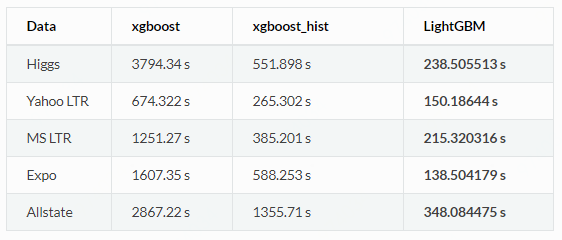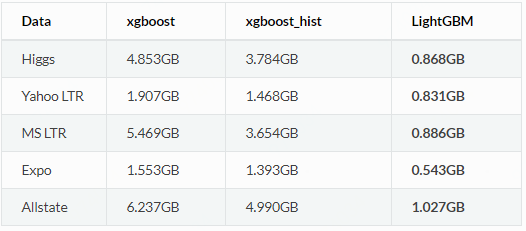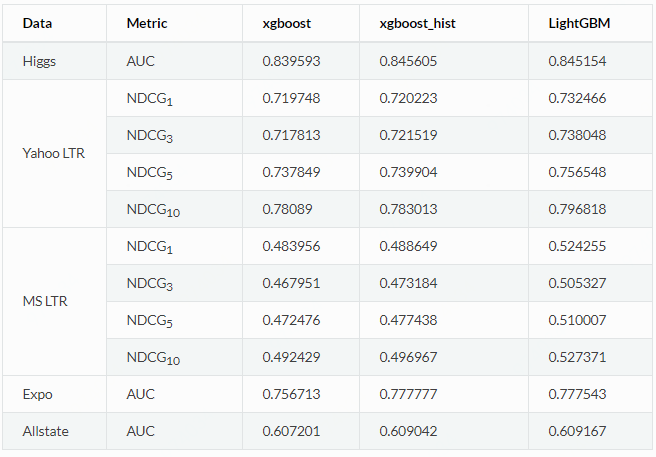# 二、LightGBM优化

LightGBM实现了Xgboost的几乎所有功能，除GPU支持，多种应用，多种度量方式外，还做了许多的优化。

## 2.1 速度和内存的优化

xgboost中默认的算法对于决策树的学习使用基于 pre-sorted 的算法 [1, 2]，这是一个简单的解决方案，但是不易于优化。

LightGBM 利用基于 histogram 的算法 [3, 4, 5]，通过将连续特征（属性）值分段为 discrete bins 来加快训练的速度并减少内存的使用。 如下是基于 histogram 算法的优点：

• 减少分割增益的计算量
• Pre-sorted 算法需要 O(#data) 次的计算。即计算最大分裂增益需要O(#data*#features)
• Histogram 算法只需要计算 O(#bins) 次, 并且 #bins 远少于 #data。即计算最大分裂增益需要O(#bins*#features)
• 通过直方图的相减来进行进一步的加速
• 在二叉树中可以通过利用叶节点的父节点和相邻节点的直方图的相减来获得该叶节点的直方图
• 所以仅仅需要为一个叶节点建立直方图 (其 #data 小于它的相邻节点)就可以通过直方图的相减来获得相邻节点的直方图，而这花费的代价(O(#bins))很小。
• 减少内存的使用
• 可以将连续的值替换为 discrete bins。 如果 #bins 较小, 可以利用较小的数据类型来存储训练数据, 如 uint8_t。
• 无需为 pre-sorting 特征值存储额外的信息。
• 减少并行学习的通信代价

## 2.2 准确率的优化

### 2.2.1 Leaf-wise (Best-first) 的决策树生长策略LightGBM 通过 leaf-wise (best-first) 策略来生长树。它将选取具有最大 delta loss 的叶节点来生长。 当生长相同的 #leaf，leaf-wise 算法可以比 level-wise 算法减少更多的损失。

#data 较小的时候，leaf-wise 可能会造成过拟合。 所以，LightGBM 可以利用额外的参数 max_depth 来限制树的深度并避免过拟合（树的生长仍然通过 leaf-wise 策略）。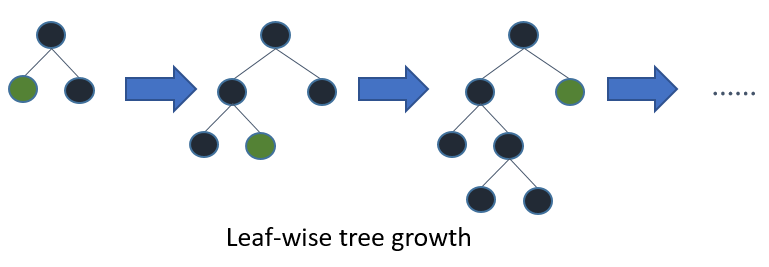## 2.3 并行学习的优化

LightGBM 提供以下并行学习优化算法：

### 2.3.1 特征并行

1. 垂直划分数据（不同的机器有不同的特征集）
2. 在本地特征集寻找最佳划分点 {特征, 阈值}
3. 本地进行各个划分的通信整合并得到最佳划分
4. 以最佳划分方法对数据进行划分，并将数据划分结果传递给其他线程
5. 其他线程对接受到的数据进一步划分

• split finding计算复杂度高为O（#data），当数据量很大的时候，慢。
• 需要对划分的结果进行通信整合，其额外的时间复杂度约为 “O（#data/8）”（一个数据一个字节）。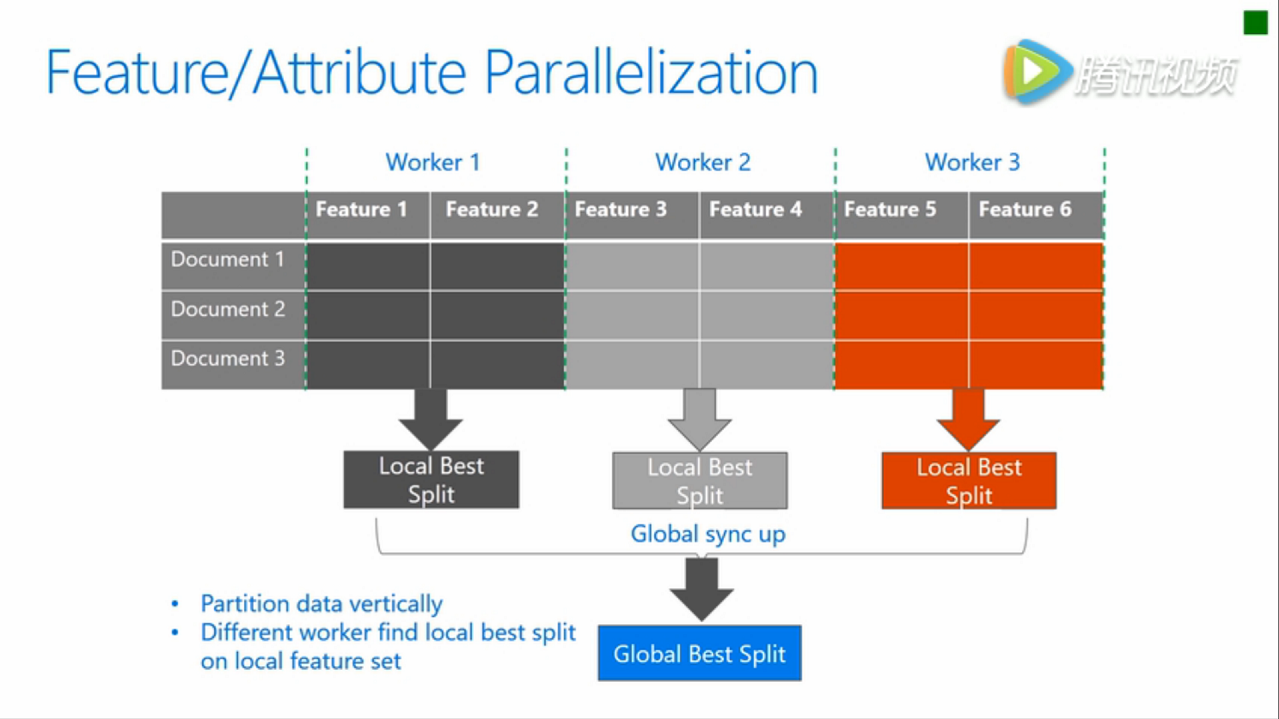LightGBM 中的特征并行

LightGBM 中特征并行的流程如下：

1. 每个线程都在本地数据集上寻找最佳划分点｛特征， 阈值｝
2. 本地进行各个划分的通信整合并得到最佳划分
3. 执行最佳划分

### 2.3.2 数据并行

1. 水平划分数据
2. 线程以本地数据构建本地直方图
3. 将本地直方图整合成全局整合图
4. 在全局直方图中寻找最佳划分，然后执行此划分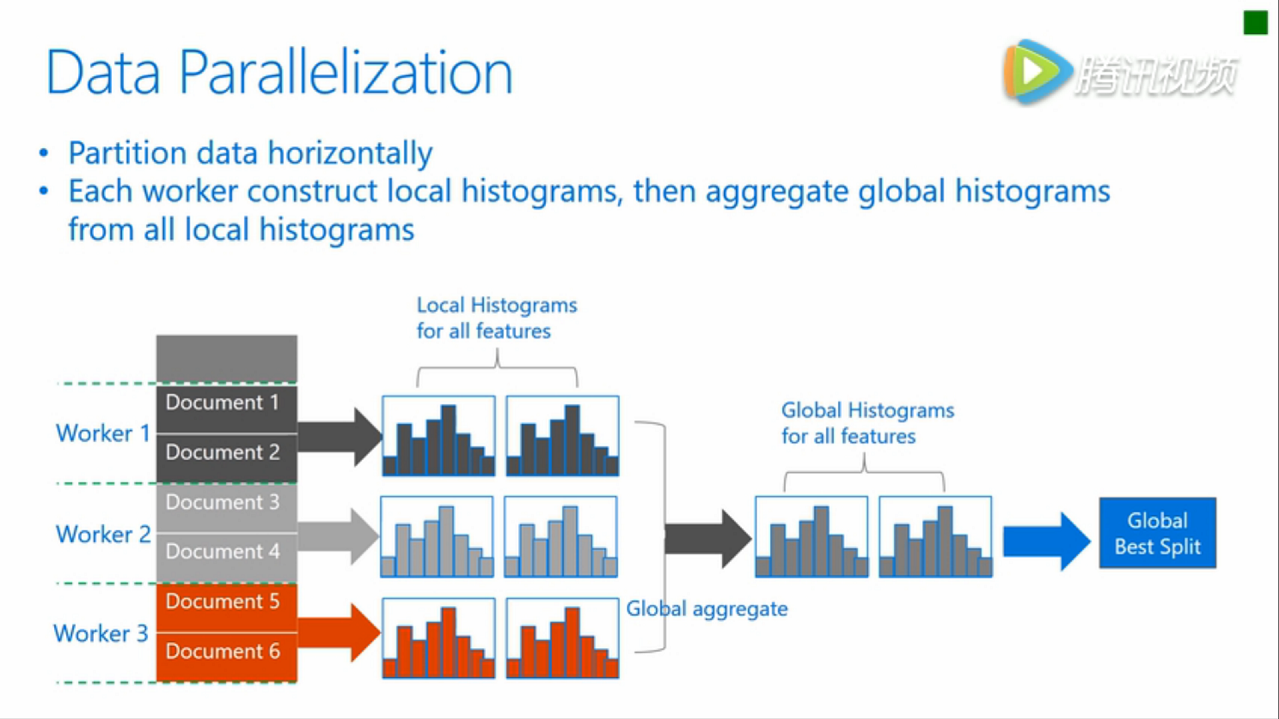• 高通讯开销。 如果使用点对点的通讯算法，一个机器的通讯开销大约为 “O(#machine * #feature * #bin)” 。 如果使用集成的通讯算法（例如， “All Reduce”等），通讯开销大约为 “O(2 * #feature * #bin)” 。

LightGBM中的数据并行

LightGBM 中采用以下方法较少数据并行中的通讯开销：

1. 不同于“整合所有本地直方图以形成全局直方图”的方式，LightGBM 使用分散规约(Reduce scatter)的方式，把直方图合并的任务分摊到不同的机器，对不同机器的不同特征（不重叠的）进行整合。 然后线程从本地整合直方图中寻找最佳划分并同步到全局的最佳划分中。
2. LightGBM 通过直方图做差法加速训练。 基于此，我们可以进行单叶子的直方图通讯，并且在相邻直方图上使用做差法。

### 2.3.3 投票并行# 三、细节Q&A

## 3.1 如果改变数据集的特征的顺序，为什么训练的结果却不一样？

A: 在LGBM中，当选择一个特征进行分裂时，如果2个特征的增益相同，那么就会选择较小索引（smaller index(id)）的特征。

## 3.2 在custom objective中Hessian的作用是什么呢？

A:在论文3.2公式（1）中，可以看到分母都是样本的个数，如果把样本的个数换成Hessian，那么就变成了Hessian最优了。

## 3.3 scale_pos_weight参数实现的机制是什么？

A:先看一下官方文档对scale_pos_weight的介绍：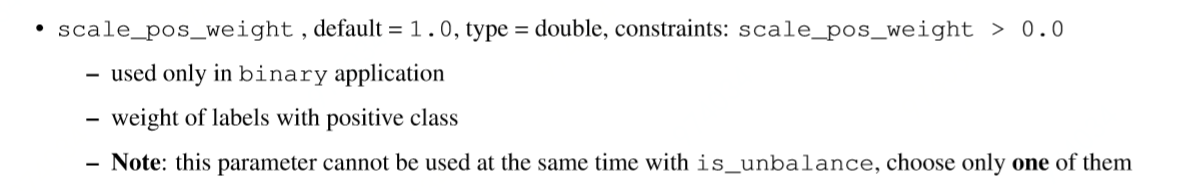number of positive samples = number of negative samples

scale_pos_weight的定义是：

sample_pos_weight = number of negative samples / number of positive samples

Related C++ code:

• xgboost proof: w += y * ((param_.scale_pos_weight * w) - w); where y is the label (0 negative or 1 positive in src/objective/regression_obj.cc)

• LightGBM proof: label_weights_ *= scale_pos_weight_; where the 2nd index (1) is for positive labels (in src/objective/binary_objective.hpp)

## 3.4 LGBM如何计算增益的？

A: 在xgb中，我们已经知道一个叶子的纯度分数为：-sum_grad / (sum_hess + lamdba)

  static double CalculateSplittedLeafOutput(double sum_gradients, double sum_hessians, double l1, double l2, double max_delta_step) {
double ret = -ThresholdL1(sum_gradients, l1) / (sum_hessians + l2);
if (max_delta_step <= 0.0f || std::fabs(ret) <= max_delta_step) {
return ret;
} else {
return Common::Sign(ret) * max_delta_step;
}
}

  static double GetSplitGains(double sum_left_gradients, double sum_left_hessians,
double sum_right_gradients, double sum_right_hessians,
double l1, double l2, double max_delta_step,
double min_constraint, double max_constraint, int8_t monotone_constraint) {
double left_output = CalculateSplittedLeafOutput(sum_left_gradients, sum_left_hessians, l1, l2, max_delta_step, min_constraint, max_constraint);
double right_output = CalculateSplittedLeafOutput(sum_right_gradients, sum_right_hessians, l1, l2, max_delta_step, min_constraint, max_constraint);
if (((monotone_constraint > 0) && (left_output > right_output)) ||
((monotone_constraint < 0) && (left_output < right_output))) {
return 0;
}
return GetLeafSplitGainGivenOutput(sum_left_gradients, sum_left_hessians, l1, l2, left_output)
+ GetLeafSplitGainGivenOutput(sum_right_gradients, sum_right_hessians, l1, l2, right_output);
}

  static double GetLeafSplitGainGivenOutput(double sum_gradients, double sum_hessians, double l1, double l2, double output) {
const double sg_l1 = ThresholdL1(sum_gradients, l1);
return -(2.0 * sg_l1 * output + (sum_hessians + l2) * output * output);
}

output就是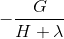；sg_l1可以认为是G。

GetSplitGains即为：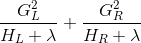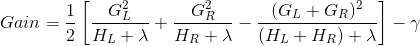void FindBestThresholdNumerical(double sum_gradient, double sum_hessian, data_size_t num_data, double min_constraint, double max_constraint, SplitInfo* output) {
is_splittable_ = false;
double gain_shift = GetLeafSplitGain(sum_gradient, sum_hessian,
meta_->config->lambda_l1, meta_->config->lambda_l2, meta_->config->max_delta_step);
double min_gain_shift = gain_shift + meta_->config->min_gain_to_split;
FindBestThresholdSequence(sum_gradient, sum_hessian, num_data, min_constraint, max_constraint, min_gain_shift, output, -1, true, false);
FindBestThresholdSequence(sum_gradient, sum_hessian, num_data, min_constraint, max_constraint, min_gain_shift, output, 1, true, false);
output->gain -= min_gain_shift;
output->monotone_type = meta_->monotone_type;
output->min_constraint = min_constraint;
output->max_constraint = max_constraint;
}

 double gain_shift = GetLeafSplitGain(sum_gradient, sum_hessian,
meta_->config->lambda_l1, meta_->config->lambda_l2, meta_->config->max_delta_step);
double min_gain_shift = gain_shift + meta_->config->min_gain_to_split;

FindBestThresholdNumerical源码中可以看到，继续调用了FindBestThresholdSequence函数。FindBestThresholdSequence被调用了两次，分别是从左到右和从右向左，类似XGBoost的缺失值自动寻找划分方向。

FindBestThresholdSequence的源码如下：

void FindBestThresholdSequence(double sum_gradient, double sum_hessian, data_size_t num_data, double min_constraint, double max_constraint, double min_gain_shift, SplitInfo* output, int dir, bool skip_default_bin, bool use_na_as_missing) {

const int8_t bias = meta_->bias;
double best_sum_left_gradient = NAN;
double best_sum_left_hessian = NAN;
double best_gain = kMinScore;
data_size_t best_left_count = 0;
uint32_t best_threshold = static_cast<uint32_t>(meta_->num_bin);

if (dir == -1) { // from right to left
.......
} else { // from left to right
double sum_left_gradient = 0.0f;
double sum_left_hessian = kEpsilon;
data_size_t left_count = 0;

int t = 0;
const int t_end = meta_->num_bin - 2 - bias;

for (; t <= t_end; ++t) {

sum_left_gradient += data_[t].sum_gradients;
sum_left_hessian += data_[t].sum_hessians;
left_count += data_[t].cnt;

data_size_t right_count = num_data - left_count;
double sum_right_hessian = sum_hessian - sum_left_hessian;
double sum_right_gradient = sum_gradient - sum_left_gradient;
// current split gain
double current_gain = GetSplitGains(sum_left_gradient, sum_left_hessian, sum_right_gradient, sum_right_hessian,
meta_->config->lambda_l1, meta_->config->lambda_l2, meta_->config->max_delta_step,
min_constraint, max_constraint, meta_->monotone_type);
// gain with split is worse than without split
if (current_gain <= min_gain_shift) continue;

// mark to is splittable
is_splittable_ = true;
// better split point
if (current_gain > best_gain) {
best_left_count = left_count;
best_sum_left_gradient = sum_left_gradient;
best_sum_left_hessian = sum_left_hessian;
best_threshold = static_cast<uint32_t>(t + bias);
best_gain = current_gain;
}
}
}

if (is_splittable_ && best_gain > output->gain) {
// update split output information
.....
}
}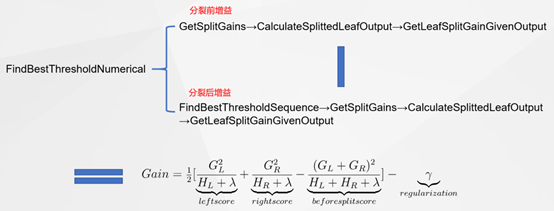## 3.5 LGBM中，回归和二分类问题中的初始第0颗树的预测值是什么？

A: 根据boost_from_average设定，使用所有标签的均值作为第0颗树的预测值。

## 3.6 当我们训练完模型后，测试集预测的值是怎么样基于训练模型得到的？

A: LGBM是类似于xgb的加法模型，在这篇文章里已经讲过了这是一个加法模型，即一个测试样本在每颗树的叶子节点的值相加就是最终预测的值。假如一共有3颗树，测试样本所在的3个树的叶子节点直接相加就是预测值。

## 3.7 LGBM如何画出决策树？

A: 与xgb画图的过程是一样的，参考链接。只是plot_tree的参数不一样。其余都是一样的。

# 获取测试集落在树模型的哪个叶子节点上
y_leaf_index = gbm.predict(X_test,  pred_leaf = True)
# 画出最后一棵树
lgb.plot_tree(gbm, tree_index=gbm.best_iteration_-1)

# 参考文献：

 Mehta, Manish, Rakesh Agrawal, and Jorma Rissanen. “SLIQ: A fast scalable classifier for data mining.” International Conference on Extending Database Technology. Springer Berlin Heidelberg, 1996.

 Shafer, John, Rakesh Agrawal, and Manish Mehta. “SPRINT: A scalable parallel classifier for data mining.” Proc. 1996 Int. Conf. Very Large Data Bases. 1996.

 Ranka, Sanjay, and V. Singh. “CLOUDS: A decision tree classifier for large datasets.” Proceedings of the 4th Knowledge Discovery and Data Mining Conference. 1998.

 Machado, F. P. “Communication and memory efficient parallel decision tree construction.” (2003).

 Li, Ping, Qiang Wu, and Christopher J. Burges. “Mcrank: Learning to rank using multiple classification and gradient boosting.” Advances in neural information processing systems. 2007.

 Shi, Haijian. “Best-first decision tree learning.” Diss. The University of Waikato, 2007.

 Walter D. Fisher. “On Grouping for Maximum Homogeneity.” Journal of the American Statistical Association. Vol. 53, No. 284 (Dec., 1958), pp. 789-798.

 Thakur, Rajeev, Rolf Rabenseifner, and William Gropp. “Optimization of collective communication operations in MPICH.” International Journal of High Performance Computing Applications 19.1 (2005): 49-66.

 Qi Meng, Guolin Ke, Taifeng Wang, Wei Chen, Qiwei Ye, Zhi-Ming Ma, Tieyan Liu. “A Communication-Efficient Parallel Algorithm for Decision Tree.” Advances in Neural Information Processing Systems 29 (NIPS 2016).